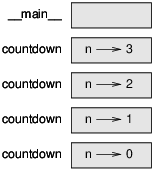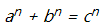# # 第五章 条件循环

## # 5.1 地板除和求模

floor除法，中文有一种翻译是地板除法，挺难听，不过凑活了，运算符是两个右斜杠：//，与传统除法不同，地板除法会把运算结果的小数位舍弃，返回整值。例如，加入一部电影的时间长度是105分钟。你可能想要知道这部电影用小时来计算是多长。传统的除法运算如下，会返回一个浮点小数：

``````    >>> minutes = 105
>>> minutes / 60
1.75
``````
1
2
3

``````    >>> minutes = 105
>>> hours = minutes // 60
>>> hours
1
``````
1
2
3
4

``````    >>> remainder = minutes - hours * 60
>>> remainder
45
``````
1
2
3

``````    >>> remainder = minutes % 60
>>> remainder
45
``````
1
2
3

## # 5.2 布尔表达式

``````    >>> 5 == 5
True
>>> 5 == 6
False
``````
1
2
3
4

True和False都是特殊的值，属于bool布尔类型；它们俩不是字符串：

``````    >>> type(True)
<class 'bool'>
>>> type(False)
<class 'bool'>
``````
1
2
3
4

``````    x != y    # x is not equal to y          二者相等
x > y    # x is greater than y          前者更大
x > y    # x is greater than y          前者更大
x < y    # x is less than y            前者更小
x >= y    # x is greater than or equal to y 大于等于
x >= y    # x is greater than or equal to y 大于等于
x <= y    # x is less than or equal to y     小于等于
``````
1
2
3
4
5
6
7

## # 5.3 逻辑运算符

n%2 == 0 或 n%3 == 0，只要条件有一个成立就是真，就是说这个可以被2或3整除就行了。

``````    >>> 42 and True
True
``````
1
2

## # 5.4 条件执行

``````    if x > 0:
print('x is positive')
``````
1
2

if后面的布尔表达式就叫做条件。如果条件为真，随后缩进的语句就运行。如果条件为假，就不运行。

if语句与函数定义的结构基本一样：一个头部，后面跟着缩进的语句。这样的语句叫做复合语句。

、复合语句中语句体内的语句数量是不限制的，但至少要有一个。有的时候会遇到一个语句体内不放语句的情况，比如空出来用来后续补充。这种情况下，你就可以用pass语句，就是啥也不会做的。

``````    if x < 0:
pass          # TODO: need to handle negative values!
``````
1
2

## # 5.5 选择执行

if语句的第二种形式就是『选择执行』，这种情况下会存在两种备选的语句，根据条件来判断执行哪一个。语法如下所示：

``````    if x % 2 == 0:
print('x is even')
else:
print('x is odd')
``````
1
2
3
4

## # 5.6 链式条件

``````    if x < y:
print('x is less than y')
elif x > y:
print('x is greater than y')
else:
print('x and y are equal')
``````
1
2
3
4
5
6

elif是『else if』的缩写。这回也还是只会有一个分支的语句会被运行。elif语句的数量是无限制的。如果有else语句的话，这个else语句必须放到整个条件链的末尾，不过else语句并不是必须有的。

``````    if choice == 'a':
draw_a()
elif choice == 'b':
draw_b()
elif choice == 'c':
draw_c()
``````
1
2
3
4
5
6

## # 5.7 嵌套条件

``````    if x == y:
print('x and y are equal')
else:
if x < y:
print('x is less than y')
else:
print('x is greater than y')
``````
1
2
3
4
5
6
7

``````    if 0 < x:
if x < 10:
print('x is a positive single-digit number.')
``````
1
2
3

``````    if 0 < x and x < 10:
print('x is a positive single-digit number.')
``````
1
2

``````    if 0 < x < 10:
print('x is a positive single-digit number.')
``````
1
2

（译者注：Python的这种友善度远远超过了C和C++，这也是为何我一直建议国内高校用Python取代C++来给本科生和研究生做编程入门课程。）

## # 5.8 递归运算

``````    def countdown(n):
if n <= 0:
print('Blastoff!')
else:
print(n)
countdown(n-1)
``````
1
2
3
4
5
6

``````    >>> countdown(3)
``````
1

n=1的时候的countdown也执行完了，返回。

n=2的时候的countdown也执行完了，返回。

n=3的时候的countdown也执行完了，返回。

（译者注：这时候一定要注意不是输出字符串就完毕了，要返回的每一个层次的函数调用者。这里不理解的话说明对函数调用的过程掌握的不透彻，一定要好好想仔细了。） 接下来你就回到主函数 main 里面了。所以总的输出会如下所示：

``````    3
2
1
Blastoff!
``````
1
2
3
4

``````    def print_n(s, n):
if n <= 0:
return
print(s)
print_n(s, n-1)
s="Python is good"
n=4
print_n(s, n)
``````
1
2
3
4
5
6
7
8

## # 5.9 递归函数的栈图Figure 5.1: Stack diagram.

## # 5.10 无穷递归

``````    def recurse():
recurse()
``````
1
2

``````    File "<stdin>", line 2, in recurse
RuntimeError: Maximum recursion depth exceeded
``````
1
2

## # 5.11 键盘输入

Python提供了内置的一个函数，名叫input，这个函数会停止程序运行，等待用户来输入一些内容。用户按下ESC或者Enter回车键，程序就恢复运行，input函数就把用户输入的内容作为字符串返回。在Python2里面，同样的函数名字不同，叫做raw_input。

``````    >>> text = input()
What are you waiting for?
>>> text
What are you waiting for?
``````
1
2
3
4

``````    >>> name = input('What...is your name?\n')
Arthur, King of the Britons!
>>> name
Arthur, King of the Britons!
``````
1
2
3
4
5

``````    >>> prompt = 'What...is the airspeed velocity of an unladen swallow?\n'
>>> speed = input(prompt)
What...is the airspeed velocity of an unladen swallow?
42
>>> int(speed)
42
``````
1
2
3
4
5
6

``````    >>> speed = input(prompt)
What...is the airspeed velocity of an unladen swallow?
What do you mean, an African or a European swallow?
>>> int(speed) ValueError: invalid literal for int() with base 10
``````
1
2
3
4

## # 5.12 调试

• 错误的类型是什么，以及

• 错误的位置在哪里。

``````    >>> x = 5
>>>  y = 6
File "<stdin>", line 1
y = 6
^
IndentationError: unexpected indent
``````
1
2
3
4
5
6

``````    import math
signal_power = 9
noise_power = 10
ratio = signal_power // noise_power
decibels = 10 * math.log10(ratio) print(decibels)
``````
1
2
3
4
5

``````    Traceback (most recent call last):
File "snr.py", line 5, in ?
decibels = 10 * math.log10(ratio)
ValueError: math domain error
``````
1
2
3
4

## # 5.13 Glossary 术语列表

floor division: An operator, denoted //, that divides two numbers and rounds down (toward zero) to an integer.

modulus operator: An operator, denoted with a percent sign (%), that works on integers and returns the remainder when one number is divided by another.

boolean expression: An expression whose value is either True or False.

relational operator: One of the operators that compares its operands: ==, !=,

, <, >=, and <=.

logical operator: One of the operators that combines boolean expressions: and, or, and not.

conditional statement: A statement that controls the flow of execution depending on some condition.

condition: The boolean expression in a conditional statement that determines which branch runs.

compound statement: A statement that consists of a header and a body. The header ends with a colon (😃. The body is indented relative to the header.

branch: One of the alternative sequences of statements in a conditional statement.

chained conditional: A conditional statement with a series of alternative branches.

nested conditional: A conditional statement that appears in one of the branches of another conditional statement.

return statement: A statement that causes a function to end immediately and return to the caller.

recursion: The process of calling the function that is currently executing.

base case: A conditional branch in a recursive function that does not make a recursive call.

infinite recursion: A recursion that doesn’t have a base case, or never reaches it. Eventually, an infinite recursion causes a runtime error.

## # 5.14 练习

### # 练习1

time模块提供了一个名字同样叫做time的函数，会返回当前格林威治时间的时间戳，就是以某一个时间点作为初始参考值。在Unix系统中，时间戳的参考值是1970年1月1号。

（译者注：时间戳就是系统当前时间相对于1970.1.1 00:00:00以秒计算的偏移量，时间戳是惟一的。）

``````    >>> import time
>>> time.time() 1437746094.5735958
``````
1
2

### # 练习2

1. 写一个函数，名叫check_fermat，这个函数有四个形式参数：a、b、c以及n，检查一下费马大定理是否成立，看看在n大于2的情况下下列等式1. 要求程序输出『Holy smokes, Fermat was wrong!』或者『No, that doesn’t work.』

2. 写一个函数来提醒用户要输入a、b、c和n的值，然后把输入值转换为整形变量，接着用check_fermat这个函数来检查他们是否违背了费马大定理。

### # 练习3

（译者注：1英寸=2.54厘米）对于任意的三个长度，有一个简单的方法来检测它们能否拼成三角形：

1. 写一个叫做is_triangle的函数，用三个整形变量为实际参数，函数根据你输入的值能否拼成三角形来判断输出『Yes』或者『No』。

2. 写一个函数来提示下用户，要输入三遍长度，把它们转换成整形，用is_triangle函数来检测这些给定长度的边能否组成三角形。

### # 4 练习4

``````    def recurse(n, s):
if n == 0:
print(s)
else:
recurse(n-1, n+s)
recurse(3, 0)
``````
1
2
3
4
5
6
1. recurse(-1, 0)这样的调用函数会有什么效果？

2. 为这个函数写一个文档字符串，解释一下用法（仅此而已）。

### # 练习5

``````    def draw(t, length, n):
if n == 0:
return
angle = 50
t.fd(length*n)
t.lt(angle)
draw(t, length, n-1)
t.rt(2*angle)
draw(t, length, n-1)
t.lt(angle)
t.bk(length*n)
``````
1
2
3
4
5
6
7
8
9
10
11Figure 5.2: A Koch curve.

### # 6 练习6

Koch科赫曲线是一种分形曲线，外观如图5.2所示。要画长度为x的这种曲线，你要做的步骤如下：

1. 画一个长度为三分之一x的Koch曲线。

2. 左转60度。

3. 画一个长度为三分之一x的Koch曲线。

4. 右转120度。

5. 画一个长度为三分之一x的Koch曲线。

6. 左转60度。

7. 画一个长度为三分之一x的Koch曲线。

1. 写一个叫做koch的函数，用一个小乌龟turtle以及一个长度length做形式参数，用这个小乌龟来画给定长度length的Koch曲线。

2. 写一个叫做snowflake的函数，画三个Koch曲线来制作一个雪花的轮廓。参考代码

3. The Koch curve can be generalized in several ways. See here for examples and implement your favorite.